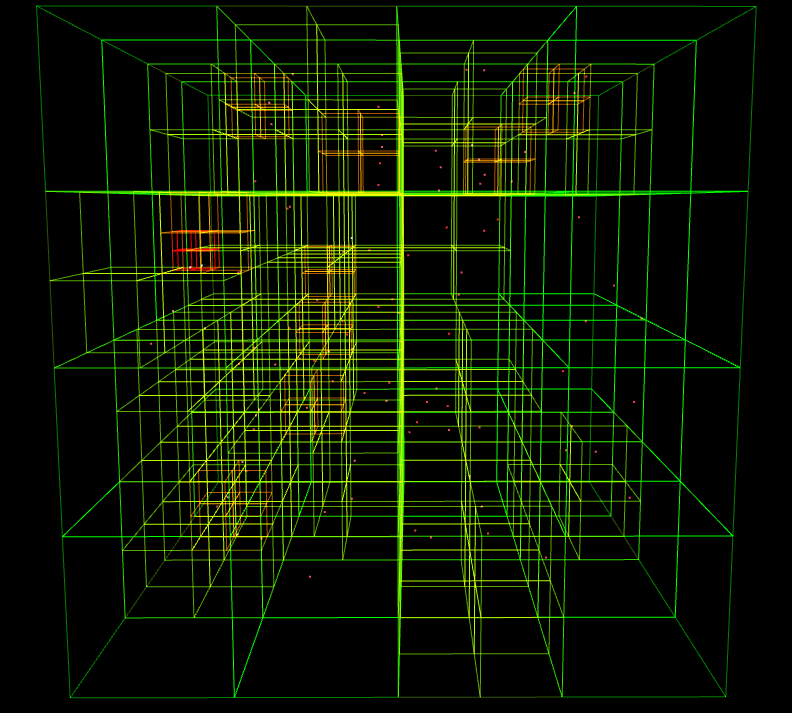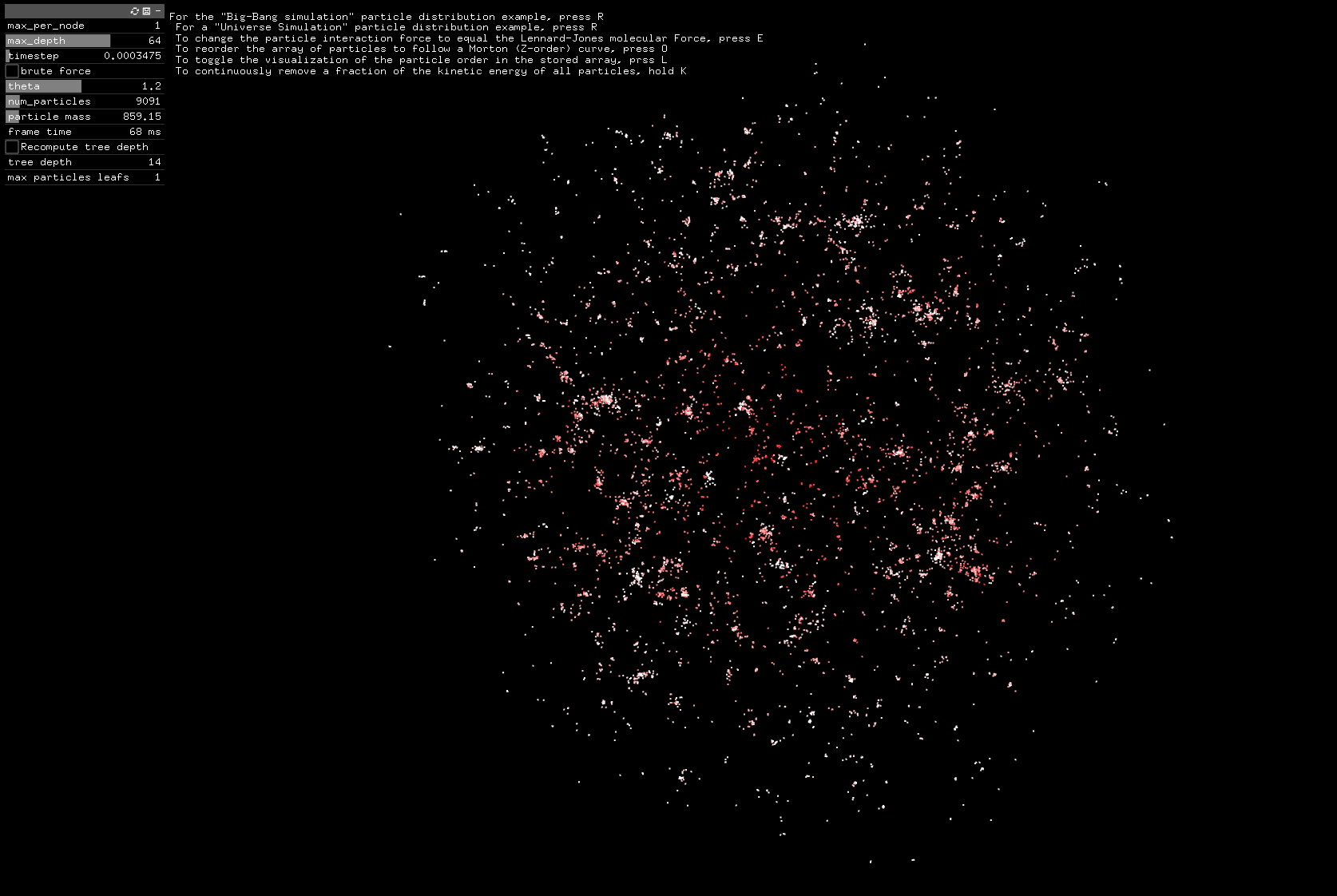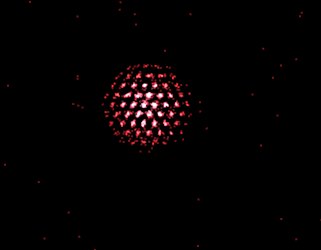# Barnes-Hut SimulationT﻿he following demo was compiled to WebAssembly using Emscripten, but is otherwise based on the same C++ code as the main project. It requires WebGL to run and is best viewed in a Desktop browser.

## What is this?

Space is cool, and as a physicist, I of course want to know how it works. In this project, let’s play god and create our own galaxy. Simulating the attractive forces between celestial bodies is a typical n-body problem that has a complexity of O(N^2). Luckily, algorithms like Barnes-hut lower the complexity to O(Nlog(N)), and extensions of it even reach O(N)

The Barnes-Hut algorithm is a hierarchical method that approximates the forces acting on each particle in a system, by approximating groups of far away particles as a single, heavy one. This allows us to simulate systems with thousands of particles, in real time.

The first step in the Barnes-Hut algorithm is to build a tree structure that divides the system into regions. This tree is constructed by recursively dividing the system into smaller sub-regions until each sub-region contains only a few particles. We use an octree, so each cuboid is divided into eight sub-segments per recursion. For a small system with just 100 particles, the tree looks like this:Once the tree structure is built, the algorithm can approximate the forces acting on each particle, by going down the tree and summing up the contributions from all other particles.

As the algorithm traverses down the branches, it checks the distance between the particle it is calculating the force on and the sub-region it is currently at. If the distance is sufficiently large, the algorithm approximates the force on the particle using the mass and and position of the sub-region as a whole (this is known as multipole expansion).

If the distance between the particle and the sub-region is not large enough, the algorithm continues to traverse down the tree, eventually reaching the leaf nodes. At this point, the algorithm degenerates into a regular brute-force computation and calculates the exact force on the particle due to all the other particles in the sub-region.

The Barnes-Hut algorithm is a highly efficient method for simulating the dynamics of galaxies. It can handle large numbers of particles with relative ease, and its hierarchical structure allows it to approximate forces with high accuracy while minimizing the number of calculations required. This makes it an ideal tool for studying the formation and evolution of galaxies, as well as other systems that exhibit complex gravitational interactions.

T﻿his project was created together with Jimmyas part of the advanced programming course in our CSE masters degree. He creates some amazing generative art, so make sure to check him out!

Formation of star and galaxy clusters from an expanding uniform initial distribution, similiar to the big-bang:T﻿he same principle can be used to simulate other n-body problems, like the ones that arise in molecular dynamics. In fact, the only thing that needs to be changed is the interaction potential, e.g. by replacing the gravitational potential with a Lennard Jones interaction. At first the particles behave like a gas, but if we “cool down” the substance by continuously removing energy, the particles settle into an energetic equilibrium after a while, forming a “crystal lattice”. This form of idealized matter is often called Lennard Jones fluid.